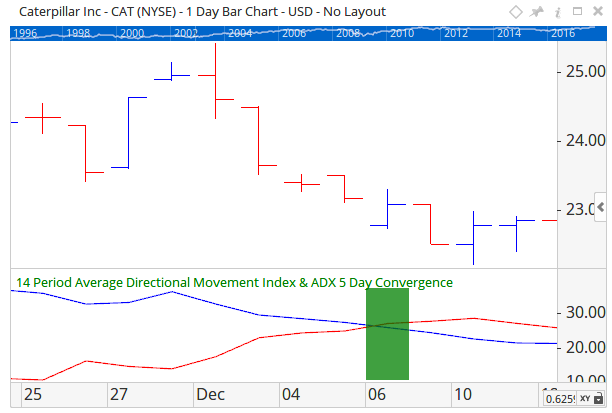# Vergence

Author: Optuma Team Last updated: Oct 19, 2017 11:23

## Overview

The VERGENCE() scripting function is used to find divergence or convergence between two plots. For example, find the signal where two moving averages are considered Diverged (moving apart) for the last 3 bars.

The script to find the above example would be…

MA1 = MA(BARS=20);
MA2 = MA(BARS=50);
VERGENCE(MA1, MA2, LOOKBACK=3, TYPE=Divergence)

You can see in the above script Lines 1 and 2 set the two plots to be used in the Vergence function.

On the chart it would look like this:Another example, find the signal when the ADX DM+ Line converges with the ADX DM- line for 5 consecutive days.